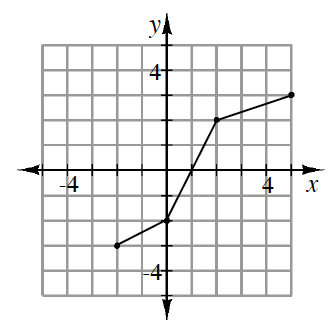### Home > A2C > Chapter 6 > Lesson 6.1.3 > Problem6-45

6-45.

The function $f(x)$ is represented in the graph below. Draw a graph of its inverse function. Be sure to state the domain and range for both $f(x)$ and $f^{−1}(x)$.Draw the line $y=x$ and then reflect $f(x)$ across that line, or reverse the coordinates of the points.

For $f(x)$ the domain is: $−2\le x\le5$, and the range is: $−3\le y\le3$.

Use the eTool below to graph the inverse function.
Click the link at right for the full version of the eTool:  6-45 HW eTool